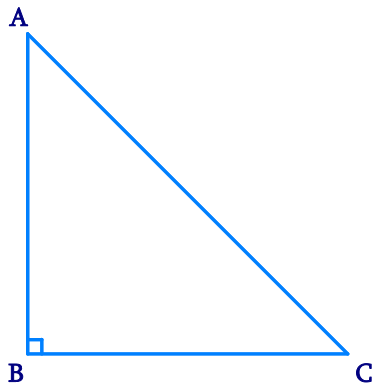# Ex.7.4 Q1 Triangles Solution - NCERT Maths Class 9

Go back to  'Ex.7.4'

## Question

Show that in a right angled triangle, the hypotenuse is the longest side.

Video Solution
Triangles
Ex 7.4 | Question 1

## Text Solution

What is Known?

A right-angled triangle.

To prove:

Hypotenuse is the longest side.

Reasoning:

In a triangle if one angle is of $$90$$ degree then the other two angles have to be acute and we can use the fact that in any triangle, the side opposite to the larger (greater) angle is longer.

Steps:Let us consider a right-angled triangle $$ABC$$, right-angled at $$B$$. In $$\Delta ABC$$,

\begin{align} & \angle A + \angle B + \angle C = 180^{\circ} \\&(\text{Angle sum property of a triangle})\\\\ & \angle A + 90^{\circ} + \angle C = 180^{\circ}\\ & \angle A + \angle C = 90^{\circ} \end{align}

Hence, the other two angles have to be acute (i.e., less than $$90^{\circ}$$). $$\angle B$$ is the largest angle in $$\Delta ABC$$.

$$\angle B \gt \angle A$$  and  $$\angle B \gt \angle C$$

$$AC \gt BC$$  and  $$AC \gt AB$$

[ In any triangle, the side opposite to the larger (greater) angle is longer.]

Therefore, $$AC$$ is the largest side in $$\Delta ABC$$.

However, $$AC$$ is the hypotenuse of $$\Delta ABC$$.

Therefore, hypotenuse is the longest side in a right-angled triangle.

Video Solution
Triangles
Ex 7.4 | Question 1

Learn from the best math teachers and top your exams

• Live one on one classroom and doubt clearing
• Practice worksheets in and after class for conceptual clarity
• Personalized curriculum to keep up with school